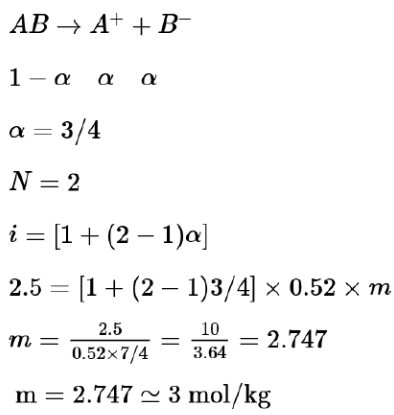# If a compound AB dissociates to the extent of 75% in an aqueous solution,Question:

If a compound $\mathrm{AB}$ dissociates to the extent of $75 \%$ in an aqueous solution, the molality of the solution which shows a $2.5 \mathrm{~K}$ rise in the boiling point of the solution is_________________  molal. (Rounded-off to the nearest integer)

$\left\lceil K_{b}=0.52 \mathrm{~K} \mathrm{~kg} \mathrm{~mol}^{-1}\right]$

Solution:

(3)